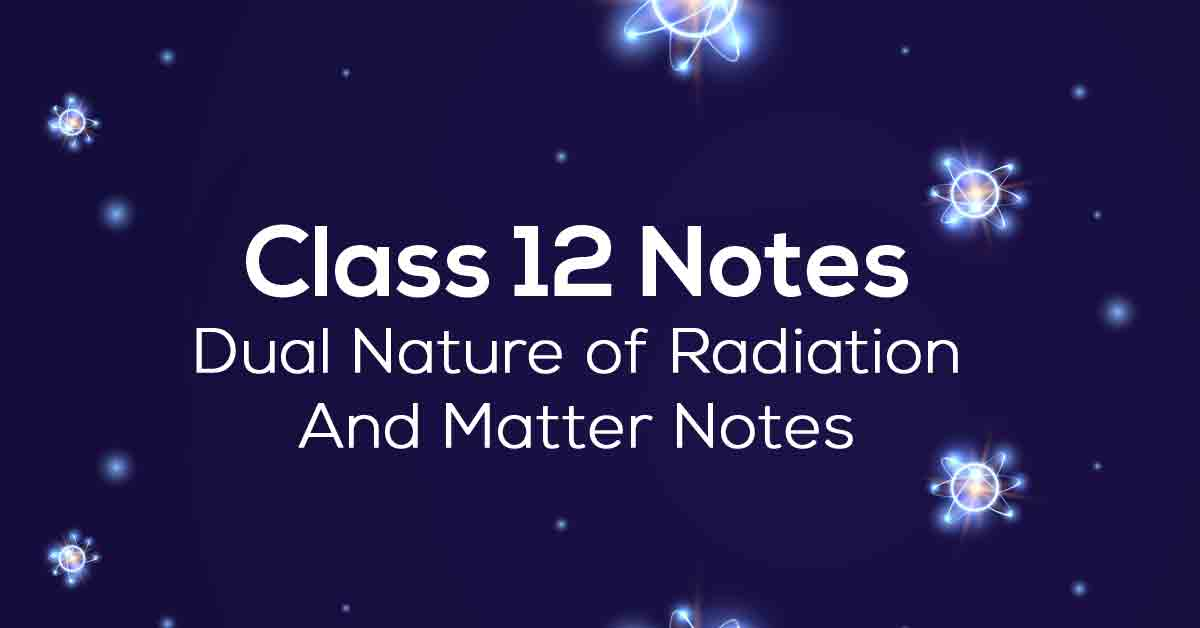Class 12th Physics Dual nature Of Radition and Matter NCERT Notes CBSE 2023

# Dual Nature of Radiation and Matter Class 12 Notes# Chapter 11 Dual Nature of Radiation and Matter

In Chapter 11 of class 12 Physics, students learn about the dual nature of radiation and matter. Students can use NCERT notes 12th Physics Chapter 11 to better understand concepts by doing text exercises. Students can follow the solutions to understand the correct approach to answering the questions in the text. Scroll down to learn more about the Chapter 11 Physics Class 12 NCERT notes.

### Points to Remember

We have provided some important points that are covered in NCERT Class 12 Physics Chapter 11 to help students in their exam preparations. Refer to the list below:

• The minimum energy required by an electron to escape from the metal surface is called the work function of the metal.

• One electron volt is the energy gained by an electron when it has been accelerated by a potential difference of 1 Volt so that 1eV = 1.602 x 10−19 J.

• There are three ways to provide energy to an electron so that it escapes the metal surface: Thermionic emission, Field emission, Photoelectric emission.

• Some photosensitive substances emit electrons called photoelectrons when they are illuminated by light by a phenomenon called the photoelectric effect. A stream of photoelectrons is called a photocurrent.

• The minimum frequency of the incident photon for the photoelectric effect to happen is called threshold frequency, and the energy of the photon at this frequency is equal to the work function of the metal.

• According to Heisenberg’s uncertainty principle, it is not possible to measure both the position and momentum of a particle at the same time exactly. The uncertainty in position (Δx) and in momentum (ΔP) are related as ΔxΔP = h/2π.

• Discovery of diffraction of electrons by C.J. Davisson and L.H. Germer lead to verification of the wave nature of electrons. In this experiment, electrons are bombarded on a metal that scatters them. The scattered electrons are collected by a collector and it is observed that the intensity of the scattered electrons is dependent on the subtle variations in the position of the collector, proving the phenomenon of diffraction in electrons.

### Topics and Sub- topics

In this chapter, students learn about radiation, the duality of matter, and the work function of metals. The minimum energy required for an electron to be ejected from a metal surface is called the metal's work function. The energy (greater than the work function (φο)) required to eject electrons from the metal surface can be provided by moderate heating, application of a strong electric field, or irradiation with light of the appropriate frequency.

Before going into the details of this article, students can refer to the table below to identify all the important topics covered in this chapter.

 Exercise Number Topics Description Ex 11.1 Electron Emission Ex 11.2 Photoelectric Effect Ex 11.3 Einstein’s Equation of Photoelectric Effect Ex 11.4 Photoelectric Effect and Wave Theory of Light Ex 11.5 Particle Nature Of Light: The Photon Ex 11.6 Wave Nature Of Matter Ex 11.7 Davisson-Garner Experiment

### Few Important Questions

• What is ‘Photoelectric effect?

The phenomenon of emission of electrons from the surface of the metal when the light of suitable frequency falls on it is called the photoelectric effect.

• What is a ‘Magnetic field’?

The portion of space near a magnetic body or a current-carrying body in which the magnetic forces due to the body or current can be detected is called a ‘Magnetic field’.

• What is ‘De Broglie's equation?

λ = h/mv, where λ is the wavelength, h is Planck’s constant, and m is the mass of a particle, moving at a velocity v. de Broglie suggested that particles can exhibit properties of waves.

### Practice Questions

1. 3 V is The photoelectric cut-off voltage in a certain experiment. Calculate the maximum kinetic energy of photoelectrons emitted.

2. Find the

1. maximum frequency, and

2. 50 kV electrons produce a minimum wavelength of X-rays.

1. Monochromatic light of wavelength 700 nm is produced by a helium-neon laser. The power emitted is 10 mW.

1. The momentum and energy of each photon in the light beam are?

2. Photons arrive at a target irradiated by this Beam. Find out the number of those photons (Assume the beam to have a uniform cross-section that is less than the target area), and

3. In order to have the same momentum as that of the photon, how fast does a hydrogen atom have to travel?